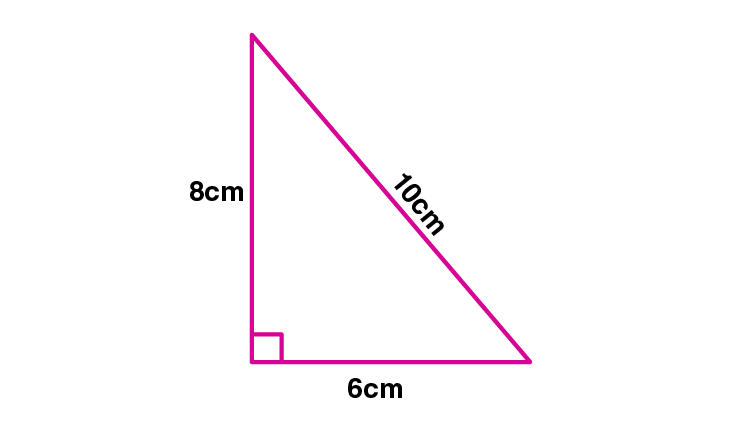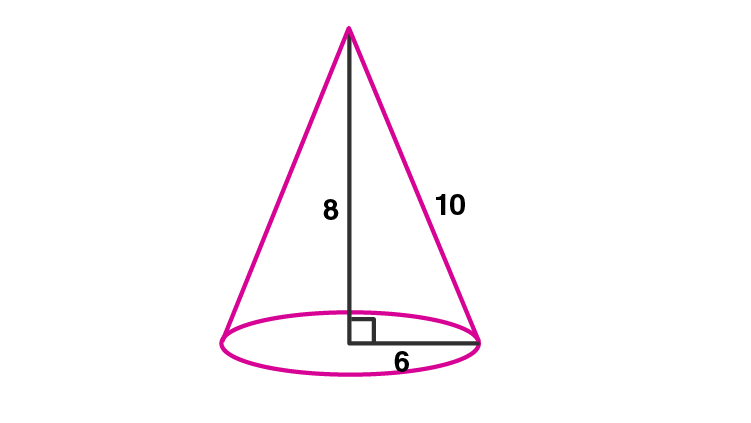Newbie

# A right triangle with sides 6 cm, 8 cm and 10 cm is revolved about the side 8 cm. Find the volume and the curved surface of the cone so formed. (Take pi = 3.14)

• -1

M.L Aggarwal book Important Question of class 10 chapter Based on Mensuration for ICSE BOARD.
A right triangle with sides given is revolved about a side .
Find the volume and the curved surface of the cone so formed
This is the Question Number 17, Exercise 17.2 of M.L Aggarwal.

Share

1.The triangle is rotated about the side 8cm.So the height of the resulting cone, h = 8 cm

Radius, r = 6 cm

Slant height, l = 10 cm

Volume of the cone, V = (1/3)r2h

V = (1/3)×3.14×62×8

V = (1/3)×3.14×36×8

V = 3.14×12×8

V = 301.44 cm3

Hence the volume of the cone is 301.44 cm3.

Curved surface area of the cone = rl

= 3.14×6×10

= 188.4

Hence the curved surface area of the cone is 188.4 cm2.

• 0